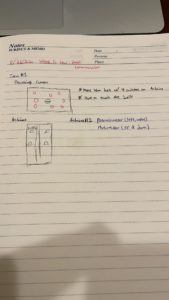# Week 10: Mini Game for Arduino & Processing Serial Communication

IDEA

For this week’s assignment, I could not really think of another creative or original game so I just decided to create a simpler version of the game that I had created for my Midterm project but with a little twist. That twist is that instead of controlling the user ball with only arrow keys, I made it available to control the left & right movement of the ball with a potentiometer on the Arduino along with two buttons (Red and Green buttons) that controls the up and down movement of the user ball, Red being up and Green being down.Challenges/Difficulties

There was not as much difficulties faced throughout the process of making it, but I would say that the most difficult part I encountered was understanding the relationship between Arduino and Processing as I was not able to fully grasp all of the information from our last lab session. However, after talking with Professor Aaron, I was able to get a clearer idea of serial communication.

Final Demonstration

Arduino Code

```const int greenSwitch = 3;
const int redSwitch = 4;

void setup() {
// put your setup code here, to run once:
Serial.begin(9600);
Serial.println("0,0");
pinMode(greenSwitch, INPUT);
pinMode(redSwitch, INPUT);
}

void loop() {
if (Serial.available() > 0) {
delay(1);
Serial.print(sensor);
Serial.print(',');
Serial.print(sensor2);
Serial.print(',');
Serial.println(sensor3);
}
}```

Processing Code

```import processing.serial.*;
Serial myPort;
int xPos=0;
Balls [] balls;
//Timer timer;
userBall myBall;
int numberofballs = 15;
int amountcollided;
void setup() {
size(1280, 960);
//printing out all the available ports
printArray(Serial.list());
//make the chosen port from the list as a string or a name
String portname=Serial.list();
/*initializes the class "Serial"
the 9600 is the baud (kinda the rate that bytes are being uploaded or transferred
*/
myPort = new Serial(this, portname, 9600);
//timer = new Timer();
myBall = new userBall();
balls = new Balls [numberofballs];
for (int i=0; i<balls.length; i++) {
balls[i] = new Balls();
}
}
void draw() {
background(255);
//fill(0);
//ellipse(xPos, height/2, 50, 50);
for (int i=0; i<balls.length; i++) {
balls[i].drawBalls();
}
myBall.drawuserBall();
myBall.checkEdge();
collisionDetection();
//timer.showTimer();
}

void serialEvent(Serial myPort) {
s=trim(s);
//if there's sth in s
if (s!=null) {
//split the string s based on commmas and put it into integer array "values"
int values[]=int(split(s, ','));
/*as long as there's a total of three arrays of information from Arduino
*/
if (values.length==3) {
//potentiometer value
myBall.xPos=values;
//sthis is values for greenswitch
if (values ==1) {
myBall.yPos +=3;
}
//this is values for redswitch
if (values == 1) {
myBall.yPos -=3;
}
myBall.xPos = int (map(myBall.xPos, 0, 1023, 0, width));
}
}
//println(xPos);
myPort.write('0');
}

void collisionDetection() {
amountcollided = 0;
for (int i=0; i<balls.length; i++) {
//if the x and y location distance of any NPC ball and user ball added together is smaller than the radius of the NPC ball and user ball, then it means it has collided
//println(dist(balls[i].locx, balls[i].locy, myBall.locx, myBall.locy));
//balls[i].iscollided=true; //if it collides, changes the boolean to true, changing the image
//if (balls[i].iscollided == true) {
//  amountcollided +=1;
stroke(255);
fill(139,0,139);
ellipse(balls[i].locx, balls[i].locy, balls[i].ballwidth, balls[i].ballheight);
//}
}
}
}```
```class Balls {
float locx, locy;
float ballwidth, ballheight;
boolean iscollided=false;

Balls() {
locx=random(width); //putting random will let the balls appear at different locations
locy=random(height);
}
void drawBalls() {
stroke(166, 134, 230);
fill(255, 0, 0);
ellipse(locx, locy, ballwidth, ballheight);

}
void collided() {
if (iscollided == true) {
stroke(255);
fill(255);
ellipse(locx, locy, ballwidth, ballheight);
}
}
}```
```class Timer {
float locx, locy;
float timerwidth, timerheight;
int countdown=20;
int seconds;
float startTime;

Timer() {// ask about the logic behind this and how to create a countdown timer?
textSize(50);

startTime= millis()/1000 + countdown;
seconds = int(startTime- millis()/1000);
}
void showTimer() {
if (seconds<0) {
startTime= millis()/1000 + countdown;//resets the timer and restarts it when less than 0
//if seconds is zero, display "game over"
} else {
seconds = int(startTime- millis()/1000);
fill(255, 0, 0);
text(seconds, width/2-100, 100);
}
}
}```
```class userBall {
float xPos, yPos;
float ballwidth, ballheight;
//float xspeed=5;
//float yspeed=5;

userBall() {//the constructor for this userBall object
//xPos=width/2;
xPos = 0;
yPos=0;
}
void drawuserBall() {
fill(0);
stroke(0, 0, 255);
ellipse(xPos, yPos, ballwidth, ballheight);
}
void checkEdge() {
if (yPos < 0) {
yPos = height;
}
if (yPos >height){
yPos = 0;
}
}
}```

Posted on Categories Fall 2020